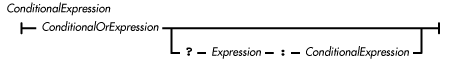start page | rating of books | rating of authors | reviews | copyrights

### 4.12 Conditional Operator

The conditional operator (? :) is a ternary operator. The operator selects one of two expressions for evaluation, based on the value of its first operand. In this way, the conditional operator is similar to an if statement. A conditional operator may appear in a conditional expression:The conditional operator produces a pure value. Conditional expressions group from right to left. Consider the following expression:

```g?f:e?d:c?b:a
```

It is equivalent to

```g?f:(e?d:(c?b:a))
```

The first operand of the conditional operator must be of type boolean, or a compile-time error occurs. If the first operand evaluates to true, the operator evaluates the second operand (i.e., the one following the ?) and produces the pure value of that expression. Otherwise, if the first operand evaluates to false, the operator evaluates the third operand (i.e., the one following the :) and produces the pure value of that expression. Note that the conditional operator evaluates either its second operand or its third operand, but not both.

The second and third operands of the conditional operator may be of any type, but they must both be of the same kind of type or a compile-time error occurs. If one operand is of an arithmetic type, the other must also be of an arithmetic type. If one operand is of type boolean, the other must also be of type boolean. If one operand is a reference type, the other must also be a reference type. Note that neither the second nor the third operand can be an expression that invokes a void method.

The types of the second and third operands determine the type of pure value that the conditional operator produces. If the second and third operands are of different types, the operator may perform a type conversion on the operand that it evaluates. The operator does this to ensure that it always produces the same type of result for a given expression, regardless of the value of its first operand.

If the second and third operands are both of arithmetic types, the conditional operator determines the type of value it produces as follows:

• If both operands are of the same type, the conditional operator produces a pure value of that type.

 Some of these rules are different from the way it is done in C/C++. In those languages, integer data of types smaller than int are always converted to int when they appear in any expression.

• If one operand is of type short and the other operand is of type byte, the conditional operator produces a short value.

• If one operand is of type short, char, or byte and the other operand is a constant expression that can be represented as a value of that type, the conditional operator produces a pure value of that type.

• Otherwise, if either operand is of type double, the operator produces a double value.

• Otherwise, if either operand is of type float, the operator produces a float value.

• Otherwise, if either operand is of type long, the operator produces a long value.

• Otherwise, if either operand is of type int, the operator produces an int value.

If the second and third operands are both of type boolean, the conditional operator produces a pure boolean value.

If the second and third operands are both reference types, the conditional operator determines the type of value it produces as follows:

• If both operands are null, the conditional operator produces the pure value null.

• Otherwise, if exactly one of the operands is null, the conditional operator produces a value of the type of the other operand.

• Otherwise, it must be possible to cast the value of one of the operands to the type of the other operand, or a compile-time error occurs. The conditional operator produces a value of the type that would be the target of the cast.

References Arithmetic Types; Boolean Type; Boolean OR Operator ||; Expression 4; Order of Operations; Reference Types